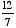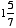index: click on a letter A B C D E F G H I J K L M N O P Q R S T U V W X Y Z A to Z index index: subject areas numbers & symbols sets, logic, proofs geometry algebra trigonometry advanced algebra & pre-calculus calculus advanced topics probability & statistics real world applications multimedia entrieswww.mathwords.com about mathwords website feedback

 Improper Fraction A fraction which has a larger numerator than denominator. For example,is an improper fraction. Note: Despite the name "improper," there is nothing "improper" about improper fractions. In math classes beyond Algebra I, improper fractions are usually preferred to mixed numbers such as.     See also